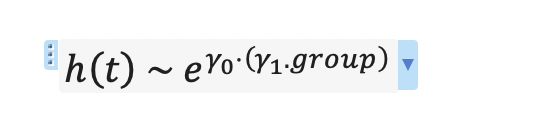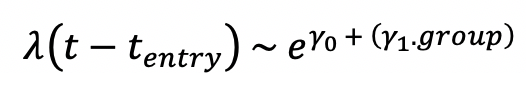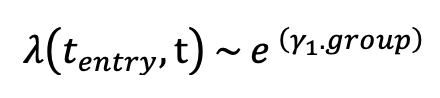# Formula for Andersen Gill extension to Cox model

Ok I am writing a paper draft where I want to include a table of equations used in different models. Some models use standard Cox models, others use the Andersen Gill counting process formulation with delayed entry.

I can represent the Cox models as follows:My question is how can I denote and Andersen Gill delayed entry model? I can’t find examples in the literature analgous to the picture.

By the way I have no clue how to render latex here but for completeness here is the latex code for that:
h\left(t\right)\ ~\ e^{\gamma_0\cdot\left(\gamma_1.group\right)}

Many thanks.

Edit: there is an error it should be gamma0 + (gamma1 * group)

i guess the appearance is the same but instead of h it’s lambda for the intensity function?

1 Like

I thought maybe so but I wasn’t 100% sure!

But then how do I depict delayed entry in the model ?

Something like this ?not sure what you mean by “delayed entry”? left censored? the distinguishing feature of AG is the extension to recurrent events.

Left truncation rather than censoring.

Basically I’m trying to write equations for this R code:

# Model 1 standard Cox
coxph(Surv(time = censor_time, event) ~ group), data=data)

# Model 2 A-G
coxph(Surv(time = entry_time, time2 = censor_time, status) ~ group), data=data)



So I thought it would be clearer for readers not so familiar with all this that the right hand side of my equations should reflect the fact that the R code has 2 time variables on its right hand side.

Edit: how about this (I realised also that gamma_0 should be there as not estimated by Cox)